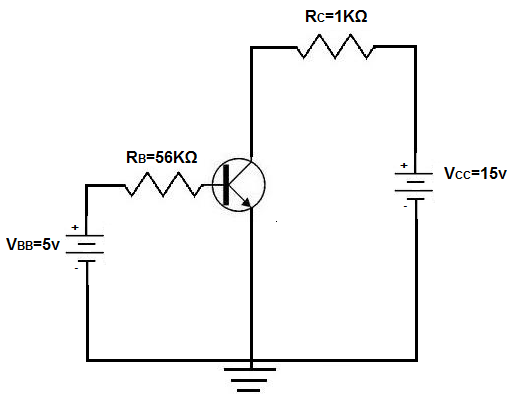﻿ Base Bias of a BJT Transistor# Base Bias of a BJT TransistorOne way to bias a BJT transistor is a method called base bias.

Base bias the simplest way to bias a BJT transistor. Base bias ensures that the voltage fed to the base, VBB, is the correct voltage, which then supplies the correct current so that the BJT has enough base current to switch the transistor on.

Below is a typical BJT receiving base bias:VBB is the base supply voltage, which is used to give the transistor sufficient current to turn the transistor on. RB is a resistance value that is used to provide the desired value of base current I B. VCC is the collector supply voltage, which is required for a transistor to have sufficient power to operate. This voltage, reverse-biases the transistor, so that the transistor has sufficient power to have an amplified output collector current. The collector resistor, RC provides the desired voltage in the collector circuit.

Using the base biasing method, the collector current IC is dependent only on the values of βdc and IB. βdc is the amplification factor by which the base current gets amplified by. So the total output current, IC will be ICdc x IB.

### Base Bias Voltage/Current Calculations

When using any biasing technique, calculations must be made of the various voltages and currents through a BJT transistor. Or else, it's impossible to tell whether the voltage and current values are correct or not.

The first calculation we will make is for the base current IB.

The base current can be found by dividing the voltage across resistor RB by the value of RB. This is shown below:

IB= (VBB - VBE)/RB

Since the voltage drop across a silicon junction is 0.7V, the value of VBE=0.7V. Therefore, IB equals:

IB= (VBB - VBE)/RB= (5v - 0.7v)/56kΩ= 76.78µA

The collector current IC can be calculated next:

ICdc x IB= 100 x 76.78µA≈ 7.68mA

With IC then known, the collector-emitter voltage, VCE can be calculated. This is shown below:

VCE= VCC - IC x RC= 15v - (7.68mA x 1KΩ)= 7.32v

Base bias can also be done with a single supply voltage, VCC, with VBB omitted. So instead of using VBB in calculations, you would just use VCC instead. The result of the calculatiosn are still the same, though.

### Disadvantages of Base Bias Method

Though base bias is one of the simplest and easiest methods to bias transistors, it is the least popular way to do so. This is because the collector current, IC, is decided by purely by the βdc of the transistor. βdc of a transistor is one of the most unstable and unpredictable parameters of a transistor. βdc can vary largely across transistors even of the same exact model and type. Therefore, base bias can lead to unpredictable actions if a transistor needs to be replaced and there are variations in the βdc of that transistor. βdc is also susceptible to changes due to temperature, as it can vary pretty largely due to ambient temperature. Base bias, then, can produce erratic circuit behavior due to transistor variations. Therefore, transistors are not commonly biased in this way.

We will explore other methods of transistor biasing including voltage-divider bias and emitter-supply bias, which provide more stability against the changes that may exist in β of a transistor.